# Geometry and Mathematical Physics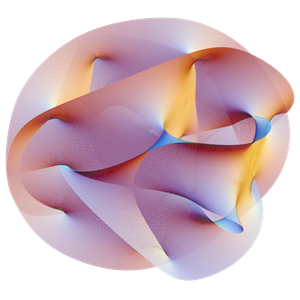Geometry plays a fundamental role in Mathematical Physics, for example it underpins several theoretical models in relativistic quantum physics, typically by encoding the underlying hidden symmetries of the model at hand. The Geometry and Mathematical Physics group at the University of Birmingham was founded in 2018 by Professor Marta Mazzocco and brings together experts in algebraic geometry, integrable systems, mirror symmetry, cluster varieties, quantum groups and quantum Teichmüller theory.

### Dr Tyler Kelly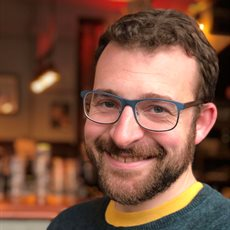Head of Geometry and Mathematical Physics

Dr Kelly is an algebraic geometer, specializing in its interactions in mathematical physics and mirror symmetry. His research primarily is focused on the question of finding a corresponding space W to a symplectic object M so that the symplectic geometry of M is encoded in the algebraic geometry W.

### Dr Cyril Closset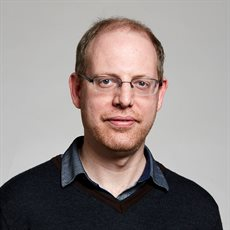Royal Society University Research Fellow (Assistant Professor)
Birmingham Fellow

The main focus of Dr Closset's research is on mathematical and 'formal' aspects of Quantum Field Theory (QFT) in the presence of supersymmetry. The underlying 'big question' is: How do we use supersymmetry to learn more about QFT at strong coupling? Along the way there are beautiful mathematical structures to be uncovered. Many of these problems arise in string theory, or have deep relations to ...

### Professor Marta MazzoccoProfessor of Mathematics

Professor Mazzocco is a specialist in the area of integrable systems, namely mathematical problems often motivated by Mathematical Physics. Her research brings geometry, quantum algebra and analysis together to describe and tackle problems which have so far resisted all other methods.

### Dr Ana Peón-Nieto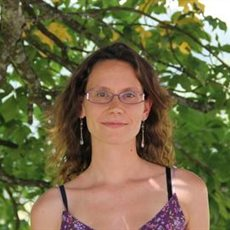Lecturer (Assistant Professor)

Dr Peón-Nieto's work focuses on Higgs bundles. These objects appeared as solutions to differential equations motivated by physics; they are to monopoles what the latter are to instantons. Several approaches allow to tackle Higgs bundles, be it from the differential, symplectic or algebraic geometric point of view.

### Dr Michel van Garrel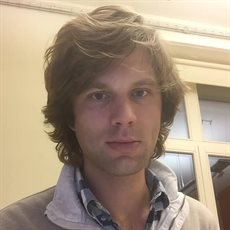Assistant Professor

Dr van Garrel specialises in enumerative geometry, in particular log Gromov-Witten theory, in birational geometry, especially with regards to rationality questions and in the Gross-Siebert programme as the mechanism that explains mirror symmetry and constructs mirror families.

## Research and Teaching Fellows

### Dr Elias Furrer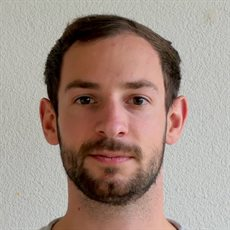Research Fellow in Mathematical Physics

Dr Furrer’s research deals with fundamental aspects and the mathematical structures of quantum field theory, gravity and string theory. He is in particularly interested in supersymmetry, dualities, topological field theory and modular forms.

### Dr Ai Guan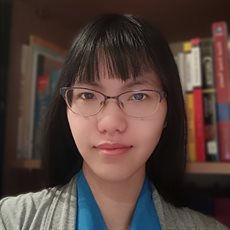Teaching Fellow

Dr Guan's research interests are in homological and homotopical algebra, derived deformation theory and category theory.

### Dr Shengyuan Huang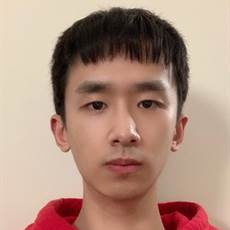Research Fellow

Dr Huang is an algebraic geometer motivated by questions in homological mirror symmetry. His previous work on orbifold Hochschild cohomology uses tools from derived geometry and has applications to mirror symmetry and hyperkähler resolution conjecture.

### Dr Omar Kidwai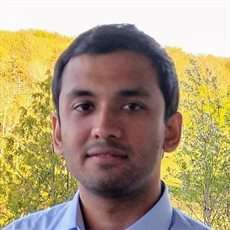Research Fellow

Dr Kidwai's research lies at the interface of algebraic geometry, differential equations, and quantum field theory.

### Dr Nikita Nikolaev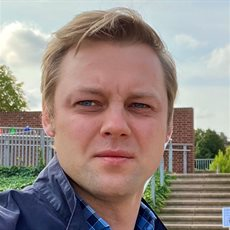Research Associate (Marie Curie Fellow)

Dr Nikolaev's research sits at the interface of algebraic geometry, differential geometry, perturbation theory, and mathematical physics, revolving around the study of moduli spaces of meromorphic connections.

### Dr Qaasim Shafi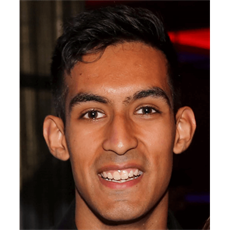Research Fellow

Dr Shafi's research is motivated by questions in Gromov-Witten theory and mirror symmetry, and utilises tools coming from intersection theory, toric geometry, logarithmic geometry and GIT.

## Visiting Professors

Image credit: The original uploader was Lunch at English Wikipedia. [CC BY-SA 2.5], via Wikimedia Commons